# Class 11 Maths NCERT Solutions for Chapter 15 Statistics Exercise 15.3### Statistics Exercise 15.3 Solutions

1. From the data given below state which group is more variable, A or B?

 Marks 10-20 20-30 30-40 40-50 50-60 60-70 70-80 Group A 9 17 32 33 40 10 9 Group B 10 20 30 25 43 15 7
Solution
Firstly, the standard deviation of group A is calculated as follows.
 Marks Group A fi Mid – point xi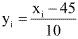yi2 fi yi fi yi2 10 – 20 9 15 -3 9 -27 81 20 – 30 17 25 -2 4 -34 68 30 – 40 32 35 -1 1 -32 32 40 – 50 33 45 0 0 0 0 50 – 60 40 55 1 1 40 40 60 – 70 10 65 2 4 20 40 70 – 80 9 75 3 9 27 81 150 -6 342
Here, h = 10, N = 150, A = 45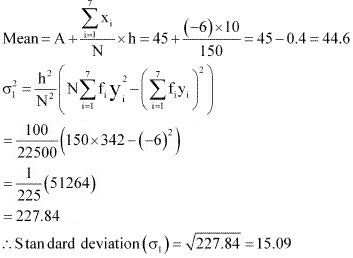The standard deviation of group B is calculated as follows.
 Marks Group A fi Mid – point xiyi2 fi yi fi yi2 10 – 20 10 15 -3 9 -30 90 20 – 30 20 25 -2 4 -40 80 30 – 40 30 35 -1 1 -30 30 40 – 50 25 45 0 0 0 0 50 – 60 43 55 1 1 43 43 60 – 70 15 65 2 4 30 60 70 – 80 7 75 3 9 21 63 150 -6 366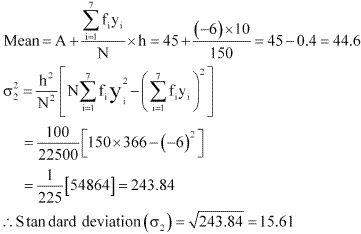Since the mean of both the groups is same, the group with greater standard deviation will be more variable.
Thus, group B has more variability in the marks.

2. From the prices of shares X and Y below, find out which is more stable in value:
 X 35 54 52 53 56 58 52 50 51 49 Y 108 107 105 105 106 107 104 103 104 101
Solution
The prices of the shares X are
35, 54, 52, 53, 56, 58, 52, 50, 51, 49
Here, the number of observations, N = 10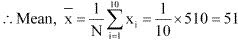The following table is obtained corresponding to shares X.
 xi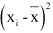35 -16 256 54 3 9 52 1 1 53 2 4 56 5 25 58 7 49 52 1 1 50 -1 1 51 0 0 49 -2 4 350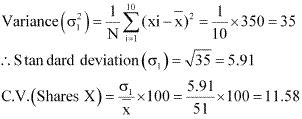The prices of share Y are
108, 107, 105, 105, 106, 107, 104, 103, 104, 101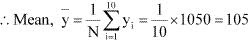The following table is obtained corresponding to shares Y.
 yi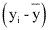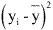108 3 9 107 2 4 105 0 0 105 0 0 106 1 1 107 2 4 104 -1 1 103 -2 4 104 -1 1 101 -4 16 40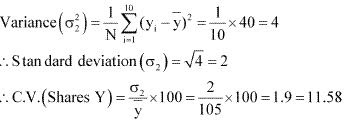C.V. of prices of shares X is greater than the C.V. of prices of shares y.
Thus, the prices of shares Y are more stable than the prices of shares X.

3. An analysis of monthly wages paid to workers in two firms A and B, belonging to the same industry, gives the following results::
 Firm A Firm B No. of wage earners 586 648 Mean of monthly wages Rs 5253 Rs 5253 Variance of the distribution of wages 100 121
(i) Which firm A or B pays larger amount as monthly wages?
(ii) Which firm, A or B, shows greater variability in individual wages?
Solution

(i) Monthly wages of firm A = Rs 5253
Number of wage earners in firm A = 586
∴Total amount paid = Rs 5253 × 586
Monthly wages of firm B = Rs 5253
Number of wage earners in firm B = 648
∴Total amount paid = Rs 5253 × 648
Thus, firm B pays the larger amount as monthly wages as the number of wage earners in firm B are more than the number of wage earners in firm A.

(ii) Variance of the distribution of wages in firm A (σ12) = 100
∴ Standard deviation of the distribution of wages in firm
A ((σ1) = √100 = 10
Variance of the distribution of wages in firm B (σ22) = 121
∴ Standard deviation of the distribution of wages in firm B (σ22) = √121 = 11
The mean of monthly wages of both the firms is same i.e., 5253. Therefore, the firm with greater
standard deviation will have more variability.
Thus, firm B has greater variability in the individual wages.

4. The following is the record of goals scored by team A in a football session:

 No. of goals scored 0 1 2 3 4 No. of matches 1 9 7 5 3
For the team B, mean number of goals scored per match was 2 with a standard deviation 1.25 goals. Find which team may be considered more consistent?
Solution
The Mean and the standard deviation of goals scored by team A are calculated as follows.
 No. of goals scored No. of matches fi xi xi2 fixi2 0 1 0 0 0 1 9 9 1 9 2 7 14 4 28 3 5 15 9 45 4 3 12 16 48 25 50 130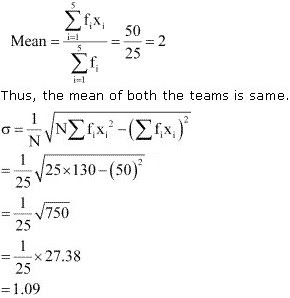The standard deviation of team B is 1.25 goals.
The average number of goals scored by both the teams is same i.e., 2. Therefore, the team with lower standard deviation will be more consistent.
Thus, team A is more consistent than team B.

5. The sum and sum of squares corresponding to length (in cm) and weight (in gm) of 50 plant products are given below: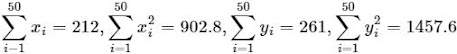Which is more varying, the length or weight?

Solution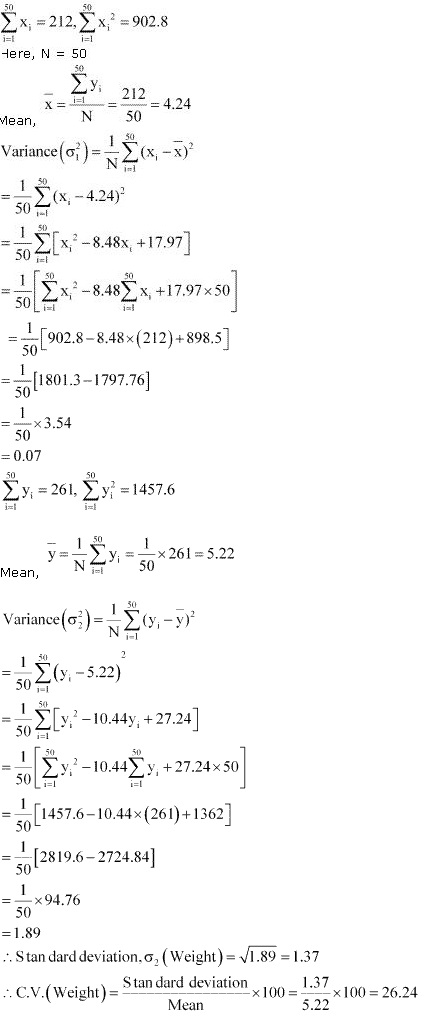Thus, C.V. of weights is greater than the c.v. of lengths. Therefore, weights vary more than the lengths.